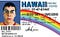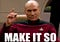1282. Group the People Given the Group Size They Belong ToSo confusing…

Introduction

Recently I’ve moved on to medium difficulty(Javascript) problems on Leetcode. I use Leet Code to measure my understanding of algorithms and data structures. I found the experience to be oddly less challenging than easy problems. Until I came across this one, wording can be confusing, and in real-life technical interviews, you’ll be able to ask for more clarity. I think it’s a problem to practice with if you’d like to focus on digesting the information given to you. Let’s dive in!

The Prompt

`There are n people that are split into some unknown number of groups. Each person is labeled with a unique ID from 0 to n - 1.You are given an integer array groupSizes, where groupSizes[i] is the size of the group that person i is in. For example, if groupSizes = 3, then person 1 must be in a group of size 3.Return a list of groups such that each person i is in a group of size groupSizes[i].Each person should appear in exactly one group, and every person must be in a group. If there are multiple answers, return any of them. It is guaranteed that there will be at least one valid solution for the given input.`

Thoughts

I know right, what’s up with this author. Let’s name a bunch of stuff that sounds the same. Tsk tsk tsk… using fancy wordplay to overwhelm the reader, for shame! No worries, let’s take a breathe and start breaking it down the line by line. Will make short work of this messy word problem with good ol’ fashion reasoning and deduction! :)

`There are n people that are split into some unknown number of groups. Each person is labeled with a unique ID from 0 to n - 1.`

Okay, the most important thing that sticks out is the unique ID from 0 to n-1. It seems pretty “mathy,” but it’s really just an index when you think about it. Let’s move on.

`You are given an integer array groupSizes, where groupSizes[i] is the size of the group that person i is in. For example, if groupSizes = 3, then person 1 must be in a group of size 3.`

Cool, groupSizes is an array of numbers. Good to know. It also makes sense of the first line… There are `n` people that are split into some unknown number of groups… As we keep reading, we see that groupSizes[i] gets you a particular group’s size(how many people are in that group). The ‘’i’ is a unique ID of a person. Thus a person with an ID of 1 is in a group the size of 3.

`Return a list of groups such that each person i is in a group of size groupSizes[i].`Grab those unique Ids 🧮

We will need to return a list of groups and their respected members by Unique Id. But how should that look like? Let’s put a pin in it for now. There is still more to read.

`Each person should appear in exactly one group, and every person must be in a group. If there are multiple answers, return any of them. It is guaranteed that there will be at least one valid solution for the given input.`

This part is straight forward. We shouldn’t have any crazy edge cases either as “It is guaranteed that there will be at least one valid solution for the given input.” Let’s go over what we know in a bulleted list.

• groupSizes is an array of integers(numbers)
• Each person is an index, that’s the unique ID
• Each group’s size is stored in the groupSizes array
• groupSizes[Person’s Id] = group size of this Person
• Each person has ONLY ONE group
• Every person MUST BE in a group
• Return ANY answers
• Each input(array of numbers) will always have AT LEAST 1 valid output

Let’s BuildYou heard the captain! 🚀

groupSizes.forEach((size, index) => {

}

My idea here is to loop through information with some variables. forEach should do the trick, as I just want to go through each group’s size value. We can break down the groupSize into its size(the value) and its index(the person’s unique id).

`let result = [], groups = {};`

Let’s make a variable set to an empty array. We will fill and return this as our answer. We will also make a variable set as an object, which will add to when we iterate.

`const groupThePeople = function(groupSizes) {  let result = [], groups = {};        groupSizes.forEach((size, index) => {        if (size in groups) { ...        `

Now will create an if the current iterated size value ALREADY EXISTS IN our group variable. If it doesn’t, let’s add it to our object as a key-value pair. The group’s size will be the key, and the values will be its members or unique ids. For now, let’s make the value an empty array. Think of it like a bucket that will hold our target ids, as we iterate.

`... if (size in groups) {       } else {          groups[size] = [index]       } ...`

Okay, here is where it gettings interesting. We want to store PRECISELY ALL of the members(unique ids) of a given group into this object. So far, when we iterate, we’ve been adding new entries, but what about the existing ones?

We will have to use more logic to get this done. Maybe if we count what we have VS. the given group size. It’s easy to access the object and compare the entries to the given size. Since we are storing in an array, why not use its length?

If we have a length LESS THAN the given size, we will push this index into our object. If not, it will simply break the if statement. It’s a lot to think about but pretty simple to write!

`if (size in groups) {            if (groups[size].length < size) {                groups[size].push(index)            }                } else {            groups[size] = [index]        }`

Let’s work on returning our answer? We will need those buckets of ids, arranged by the group’s size. Let’s check if any of those buckets are full; if they are will add them to our results array.

`...if (groups[size].length === size) {        }...`

We will use an if statement to do this, which compares the existing buckets’ length to the current iterated size. If we have a match will use a .push() array method to add the data to our results array.

`if (groups[size].length === size) {            result.push(groups[size]);        }`

One small problem, though. We will keep sending duplicate information to our result array. This isn’t very helpful, so let’s reset this groups[size] to an empty array. We won’t need this information once we’ve moved it.

`if (groups[size].length === size) {    result.push(groups[size]);                groups[size] = [];}`

Let’s return this result statement and run our code. Remember this should leave outside of our .forEach() but live inside our groupThePeople() function.

Solution

`const groupThePeople = function(groupSizes) {  let result = [], groups = {};        groupSizes.forEach((size, index) => {        if (size in groups) {            if (groups[size].length < size) {                groups[size].push(index)            }                } else {            groups[size] = [index]        }                if (groups[size].length === size) {            result.push(groups[size]);            groups[size] = [];        }    })    return result;};`

Conclusion

Pheww.. we did it! That took a bit of thinking on our end, but it was well worth it. Deciphering the wording of the problem seemed more of a challenge than thinking out the logic. Yet, It’s good practice for your overall problem-solving skills. So take the time to write down each idea, build up that list to reference later. Save that valuable in brain memory for something else.

Links

LeetCode: https://leetcode.com

## More from Sam Lesser

Career Changer, Software Engineer & Web Developer